Quantity: 0

Total: 0,00

0

# Surface area of spheres (demonstration)

### Surface area of spheres (demonstration)

The surface of a sphere consists of the set of points which are all at the same distance from a given point in space.

Mathematics

Keywords

surface of sphere, great circle, mathematics, geometry, surface

Related items

### Scenes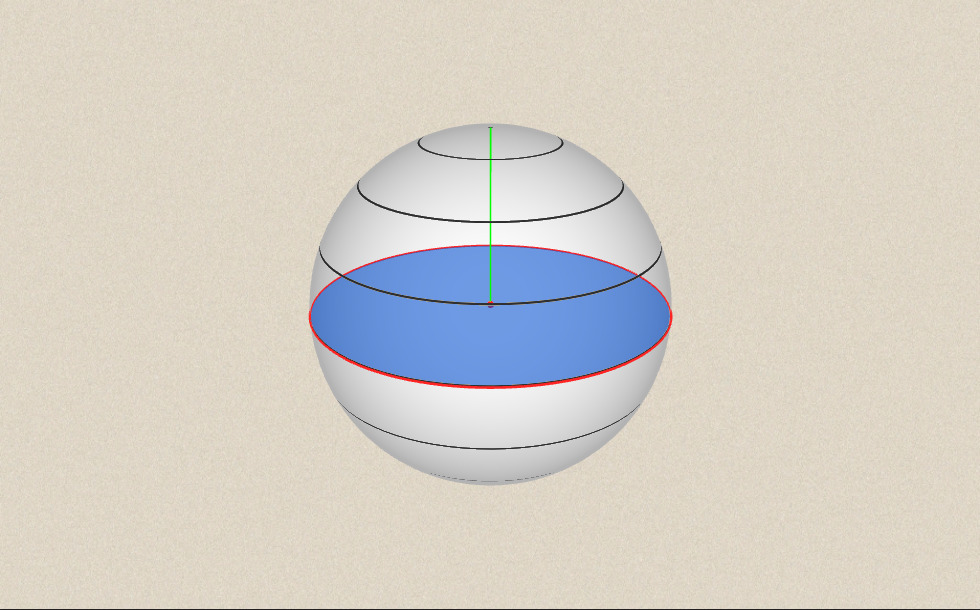### Sphere

A sphere is defined by the set of points which are all at a fixed distance (r ) from a given point in space (P ). Here r is the radius of the sphere.

If this distance is smaller than r it results in a spherical body, if it is exactly equal to r it results in a spherical surface.

The intersection of a sphere with a plane, which passes through the centre of the sphere, is called the great circle of the sphere. This is demonstrated by pressing the ´Animation´ button.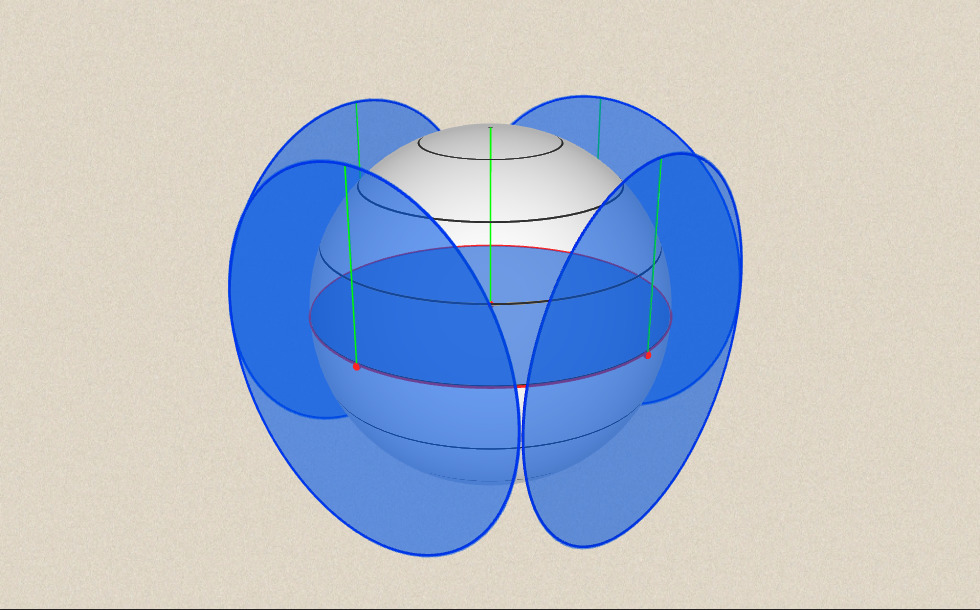### Great circles and the sphere

• great circle

The area of a great circle isand the area of the four great circles together iswhich is the formula of the surface area of the sphere.In other words, 4 great circles can cover the entire surface of the sphere. Of course, this does not work in reality, this is only a theoretical experiment.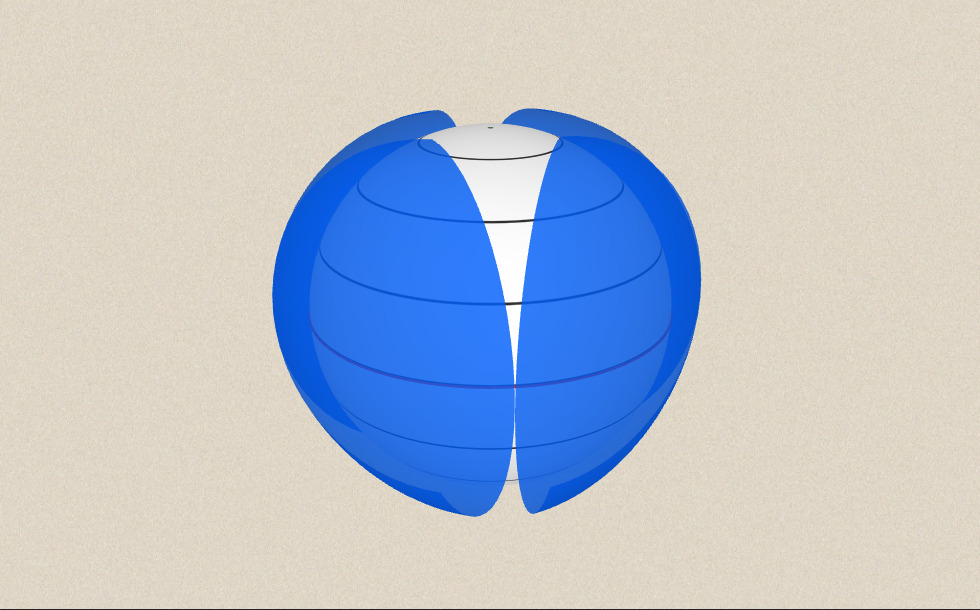### Related items#### Sphere

A sphere is the set of points which are all within the same distance from a given point in space.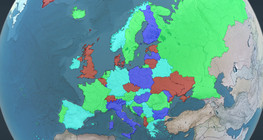#### Four colour theorem

Colour a map with the fewest number of colours possible, so that no two adjacent regions have the same colour.#### Non-orientable surfaces

The Möbius strip and the Klein bottle are special two-dimensional surfaces with only one side.#### Perimeter, area, surface area and volume

This animation presents the formulas to calculate the perimeter and area of shapes as well as the surface area and volume of solids.#### Volume of spheres (Cavalieri´s principle)

Calculating the volume of a sphere is possible using an appropriate cylinder and cone.#### Volume of spheres (demonstration)

The sum of the volume of the ´tetrahedrons´ gives an approximation of the volume of the sphere.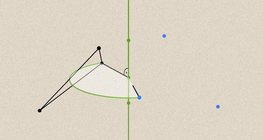#### Geometric transformations – rotation

This animation demonstrates geometric rotation, a type of geometric transformation both in plane and space.#### Solids of revolution

Rotating a geometric shape around a line within its geometric plane as an axis results in a solid of revolution.